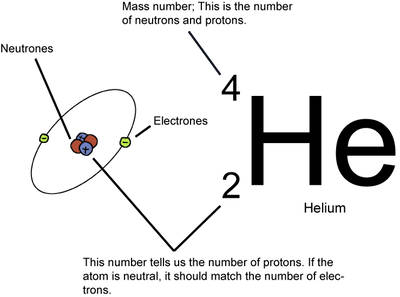Study Guide

# Atoms, Molecules, and Ions Questions

## Atoms, Molecules, and Ions Questions

1. Is the atomic weight for each element a single exact value? Please explain.

2. How is a molecule different than a compound?

3. Draw out the information that you would find for helium on a basic periodic table. Indicate what each number and symbol is and what it means.

4. Explain the difference between a molecular formula and an empirical formula. Give an example.

5. What are the three subatomic particles? Where is each located? What is each one's charge?

6. Give a brief summary of John Dalton's theory of the atom.

7. What is the Law of Conservation of Mass and what does it mean?

8. What is the uncertainty principle and what does it have to do with orbitals?

9. Explain what happens during an atomic explosion of uranium-235.

10. Explain how a blimp works and why the Hindenburg disaster happened.

### Solutions

1. Is the atomic weight for each element a single exact value? Please explain.

No, because each element can have different isotopes, meaning the number of neutrons can vary. Because each isotope has a different number of neutrons it also has a different atomic weight. The atomic weight shown on a periodic table is the average atomic weight that takes into consideration the natural abundance of each isotope.

2. How is a molecule different than a compound?

A molecule is formed when two or more atoms join together chemically. A compound is a molecule that contains at least two different elements.

3. Draw out the information that you would find for helium on a basic periodic table. Indicate what each number and symbol is and what it means.4. Explain the difference between a molecular formula and an empirical formula. Give an example.

The empirical formula is the simplest formula for a compound. A molecular formula is the same as or a multiple of the empirical formula, and is based on the actual number of atoms of each type in the compound. For example, if the empirical formula of a compound is C3H8, its molecular formula may be C3H8, C6H16, etc.

5. What are the three subatomic particles? Where is each located? What is each one's charge?

The three subatomic particles are the proton, neutron, and electron. The proton and the neutron are located inside the nucleus of the atom. The proton is positively charged and has a significant mass that is used to calculate an atom's mass number. The neutron is neutrally charged and like the proton, has a significant mass that is also a part of an atom's mass number. The electrons are located outside the nucleus inside orbitals. Electrons are negatively charged and have negligible mass. Thus, the mass of the electrons is ignored when calculating the atom's mass number.

6. Give a brief summary of John Dalton's theory of the atom.

Elements are composed of extremely small particles called atoms. All atoms of a given element are identical, having the same size, mass, and chemical properties. The atoms of one element are different from the atoms of all other elements. Compounds are composed of atoms of more than one element in whole number ratios with each other. A chemical reaction involves only the separation, combination, or rearrangement of atoms, not their creation or destruction.

7. What is the Law of Conservation of Mass and what does it mean?

This law states that matter can be neither created nor destroyed. Atoms are unchanged during a chemical reaction. If an atom, which has specific mass, exists before a reaction occurs, it also exists after a reaction occurs. This means that during the course of a reaction, mass is also conserved.

8. What is the uncertainty principle and what does it have to do with orbitals?

According to quantum theory, it's impossible to know an electron's exact position and momentum at the same time. This is known as the uncertainty principle. In other words, if you know the exact location of an electron you have zero information about its momentum. Or if you know its exact momentum, you know nothing about its location. For this reason, scientists developed the idea of orbitals, which are shapes of probability where electrons can be found at any given time.

9. Explain what happens during an atomic explosion of uranium-235.

Once uranium is enriched with U-235, it can undergo a chain reaction, in which neutrons produced by the fission of one uranium nucleus can induce fission in other uranium nuclei. The process continues until the whole sample of U-235 is gone. What is left is a whole bunch of lighter elements and a whole bunch of energy. The amount of energy produced by an atomic explosion is way more powerful than tons of dynamite and can destroy entire cities.

10. Explain how a blimp works and why the Hindenburg disaster happened.

A blimp is a very large balloon that is filled with a gas lighter than the air that surrounds it. Because it is filled with a lighter gas, the blimp floats. The Hindenburg was filled with hydrogen gas, which is lighter than air but is also flammable and highly reactive. The Hindenburg sprung a leak and the hydrogen gas caught on fire causing an explosion and fire. As a result of this accident, blimps are now filled with helium gas, which is lighter than air but is a non-reactive gas.